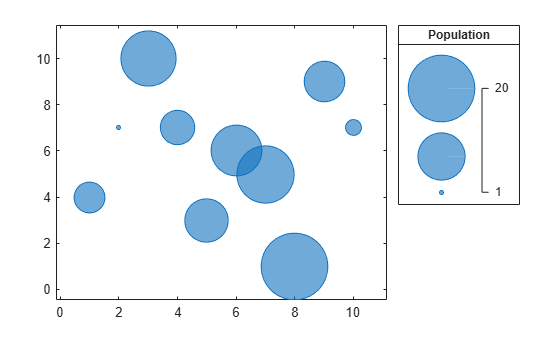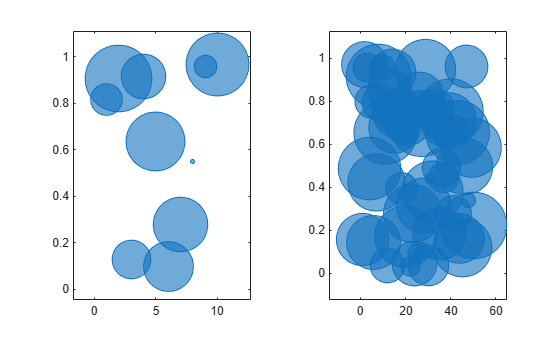# bubblesize

## 语法

``bubblesize(sizerange)``
``bubblesize(ax,sizerange)``
``sz = bubblesize``
``sz = bubblesize(ax)``

## 说明

示例

``bubblesize(sizerange)` 设置当前坐标区中气泡图的最小和最大气泡的大小。将 `sizerange` 指定为包含最小和最大直径的二元素向量，以磅为单位（1 磅 = 1/72 英寸）。`

示例

``bubblesize(ax,sizerange)` 在 `ax` 指定的坐标区中设置气泡大小。`

示例

``sz = bubblesize` 以包含最小和最大气泡直径的二元素向量形式返回当前坐标区的大小范围（以磅为单位）。`

示例

``sz = bubblesize(ax)` 返回 `ax` 指定的坐标区的大小范围。`

## 示例

全部折叠

创建一个包含图例的气泡图。

```x = 1:10; y = [4 7 10 7 3 6 5 1 9 7]; sz = [5 1 14 6 9 12 15 20 8 2]; bubblechart(x,y,sz); bubblelegend('Population','Location','northeastoutside')```获取气泡大小的当前范围。

`bubblesize`
```ans = 1×2 3 50 ```

更改气泡大小，使其直径在 `5``20` 磅之间。气泡图例会自动更新以反映新气泡大小。

`bubblesize([5 20])`创建两个数据集，然后创建一个包含两个气泡图的分块图布局。

```% Create two sets of data x1 = 1:10; x2 = 1:50; y1 = rand(1,10); y2 = rand(1,50); sz1 = randperm(10,10); sz2 = randperm(50,50); % Create tiled chart layout t = tiledlayout(1,2); % Create bubble chart in first tile ax1 = nexttile; bubblechart(x1,y1,sz1) % Create bubble chart in first tile ax2 = nexttile; bubblechart(x2,y2,sz2)```通过将 `ax2` 传递给 `bubblesize` 函数，查询右图中气泡大小的范围。

`bubblesize(ax2)`
```ans = 1×2 3 50 ```

更改右图中的气泡大小，使其直径在 `5``15` 磅之间。

`bubblesize(ax2,[5 15])`## 输入参数

全部折叠

大小范围，指定为包含正数的二元素向量，其中第二个数大于第一个数。这两个数字分别指定最小和最大气泡大小，以磅为单位（1 磅 = 1/72 英寸）。

默认情况下，气泡图的大小范围为 `[3 50]`

示例： `bubblesize([20 70])` 将最小气泡大小设置为 20 磅，将最大气泡大小设置为 70 磅。

目标坐标区，指定为 `Axes``PolarAxes``GeographicAxes` 对象。

在 R2020b 中推出